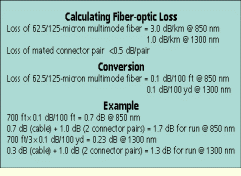# Installer Tips

A common problem in testing optical fiber for loss is determining a loss budget to compare to actual measurements made by an optical-loss test set (see "Determining optical fiber link loss," June 1995, page 52). Complicating this problem is the fact that cable-reel readings are usually in feet while loss is specified in decibels per kilometer.Convert meters to feet to simplify fiber-optic loss calculations.

Rich Tyler, Noyes Fiber Systems

Problem

A common problem in testing optical fiber for loss is determining a loss budget to compare to actual measurements made by an optical-loss test set (see "Determining optical fiber link loss," June 1995, page 52). Complicating this problem is the fact that cable-reel readings are usually in feet while loss is specified in decibels per kilometer.

Solution

This problem can be solved by converting loss values into decibels per foot or, since this would be a very small number, into decibels per 100 feet. The following example illustrates the proper conversion procedure.

Imagine you have installed a 700-foot run of 62.5/125-micron multimode fiber. The fiber is specified to have maximum losses of 3.0 dB/km at an operating wavelength of 850 nanometers and 1.0 dB/km at 1300 nm. The connectors are specified at less than 0.5 dB per mated pair. Now calculate the maximum acceptable loss for the run at each wavelength.

Procedure

1) There are 3300 feet per kilometer, so at 850 nm, the loss should be 3.0 dB/km divided by 3300 ft/km times 100, or 0.09 dB per 100 feet. This can be rounded off to 0.1 dB/100 ft.

2) Because the loss at 1300 nm is one-third the loss at 850 nm, the expected value is 0.1 dB per 300 feet.

3) An excellent rule of thumb is that 0.1 dB of loss should be expected for every 100 feet of 62.5/125-micron multimode fiber at 850 nm, and for every 100 yards of the same fiber at 1300 nm.

4) For the 700-foot run, the fiber loss at 850 nm is 7x0.1dB/ 100 ft = 0.7 dB. Add 1.0 dB for two mated pairs of connectors, and the total loss for the run is 1.7 dB at 850 nm.

5) At 1300 nm, expected fiber loss is 0.23 dB, which to be safe can be rounded up to 0.3 dB. Add the connector loss, and the total is 1.3 dB at 1300 nm.

Keep in mind that these values are calculated and not measured losses. Actual testing values may vary due to many factors, such as cable and connector quality, the expertness of terminations and the presence of splices in the cable run.Click here to enlarge image

Rich Tyler is director of marketing and sales at Noyes Fiber Systems, Laconia, NH.

More in Home
Sponsored